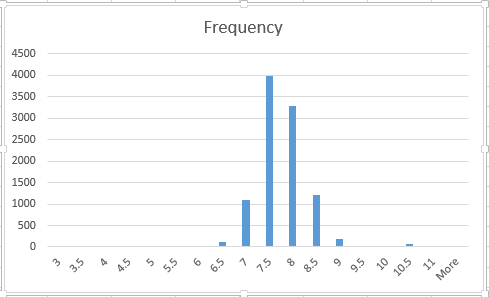# Excel: Data Transformations

Data Transformations

In statistics, data transformations are used, for lack of better words, to even out the numbers. While there are lots of reasons to perform transformations in the world of Statistics, in Data Science and Analytics, our main concern is to bring distributions to the normal form (the classic bell curve).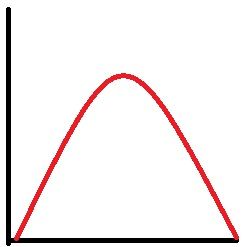As with many concepts, sometimes it is easy to see by doing. So let’s jump into Excel, you can download the file to play along here: transform1

## First things first – Install Analysis Toolkit

In order to created histograms in Excel (which we will need for this exercise), you need to install the Analysis Toolkit.

Click on the green “File” in the upper left corner of Excel, then click options.Click Add-Ins > select Analysis TookPak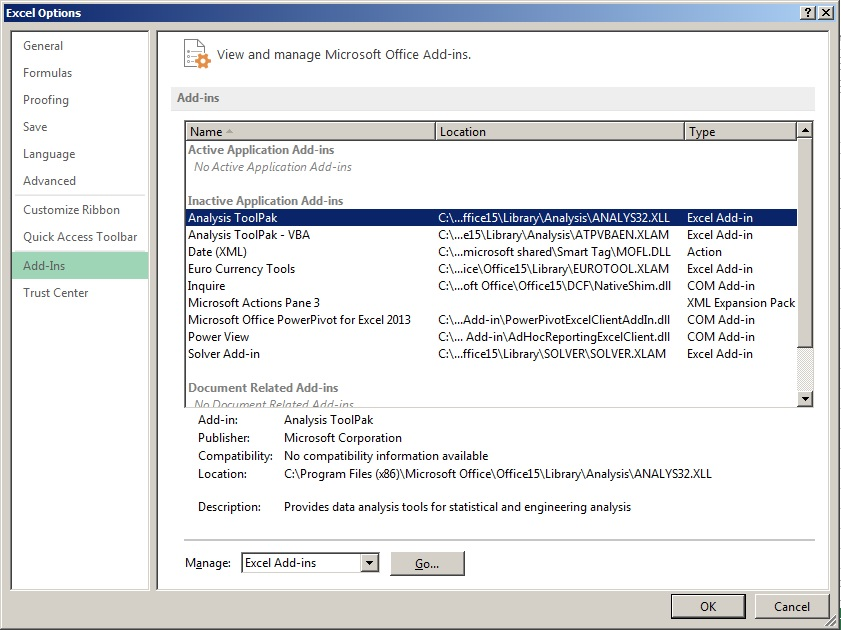Hit OK and now lets look at our data.

## The Data

We have two columns –

UNITSF =10,000 records of house square footage

Bins = list of Bin sizes we will use for the histogram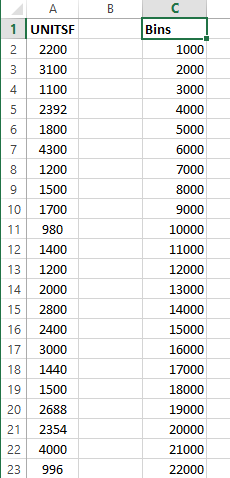First we will create a histogram to look at the data.

To do so, go to the Data tab in the Ribbon bar. Then on the far right select Data AnalysisSelect Histogram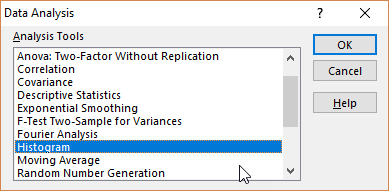For Input Range – select number values from the UNITSF column.

Bin Range – select number values from Bin column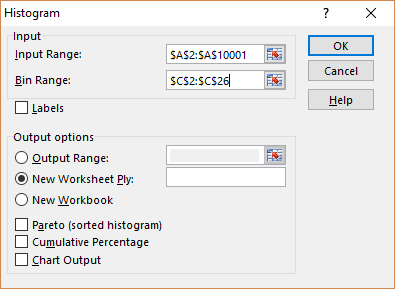Now you get a Frequency Distribution. Let’s visualize it to see better.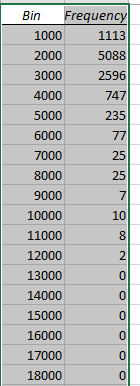In the Ribbon Bar, select Insert>Recommended Charts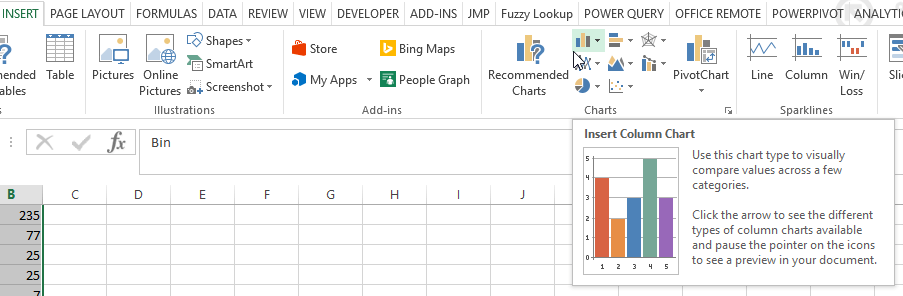Select the Frequency bar chartLook at this Frequency distribution. Notice how it bunched to the left. In Stats – they call this Right-Skewed since the long tail trails out to the right.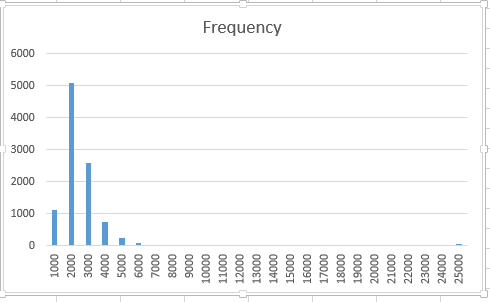The problem is, many popular data mining (machine-learning) algorithms can be easily thrown off by data like this. The algorithms (like regressions, decision trees, boosted forests, naive bayes, etc.) are designed to work better with normal distributions.

So to normalize this distribution, we have to transform the data. What that means in practice is to apply a mathematical operation to all the data in the column. Operations like: logs, square roots, cube roots, squaring, cubing, etc.

In our example, the natural log does a very good job of transforming our data.

To do so, lets start by applying the natural log to the first element in our data.

=ln(A2)Now hover over the little green box in the bottom corner until your cursor turns into a black plus sign. Double click and the natural log will be applied to all elements.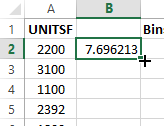Build a new bin column ranging from 3 – 11 by .5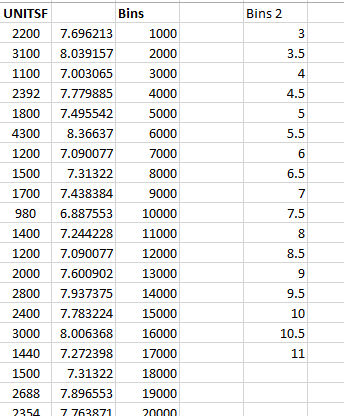Now lets build our frequency table. Data> Data Analysis > Histogram

Make sure you select the B column for Input and E column for Bin RangeNow go to your new distribution and graph it like we did before.

Notice how the new distribution looks a lot more like the class bell curve.